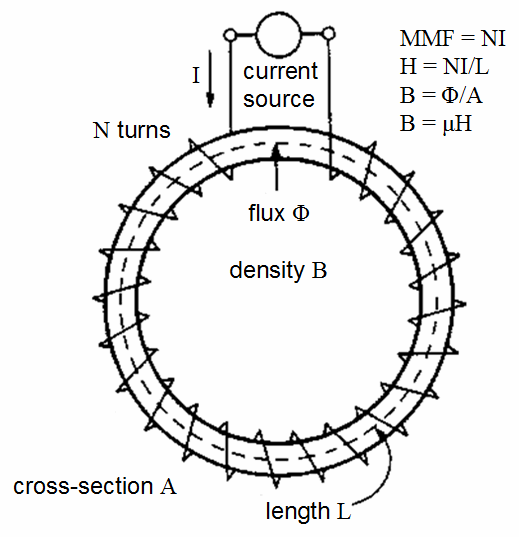Experiments with magnets and our surroundings

Twelve Fundamentals of Magnetism

6.   Magnetic Field Intensity, H [ampere-turns per meter, At/m]

http://en.wikipedia.org/wiki/Magnetic_field_intensity

b. What is it?

The Magnetic Field Intensity or Magnetic Field Strength is a ratio of the MMF needed to create a certain Flux Density (B) within a particular material per unit length of that material.c. Its unit

H = At/m, ampere-turns per meter.  Often, N is used as the number of turns of wire around a core or magnetic material.  So the H = N*I/m.

d. How is it used?

This is the horizontal axis of the B-H curve for magnetic materials, and is used to vary the magnetic flux within the material by varying the current in the solenoid, thus varying the At (varying the amperes of the ampere-turns).

To determine the B-H curve of a material, the H is varied by controlling the magnitude and polarity of the current flowing in the coil around the sample material, and the B is measured.  There are B-H graphs or charts for various types of steels and other materials used for magnetic devices.

e. An example in a vacuum

As we will see in the next section, for a vacuum, a field intensity of 796At/m (often rounded to 800At/m). will create a flux density of 1mT = 10G.